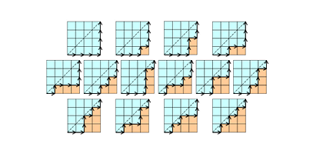# Is this a coincidence too?If we write out the decimal expansion of the smaller root of $f(x)=1000000x^2-1000000x+1$, we get the following decimal: $0.000001 \quad 000001\quad 000002\quad 000005 \quad 000014 \quad \ldots$ which we notice as the first 5 Catalan numbers. How many distinct Catalan numbers occur in a row before this pattern stops?

×